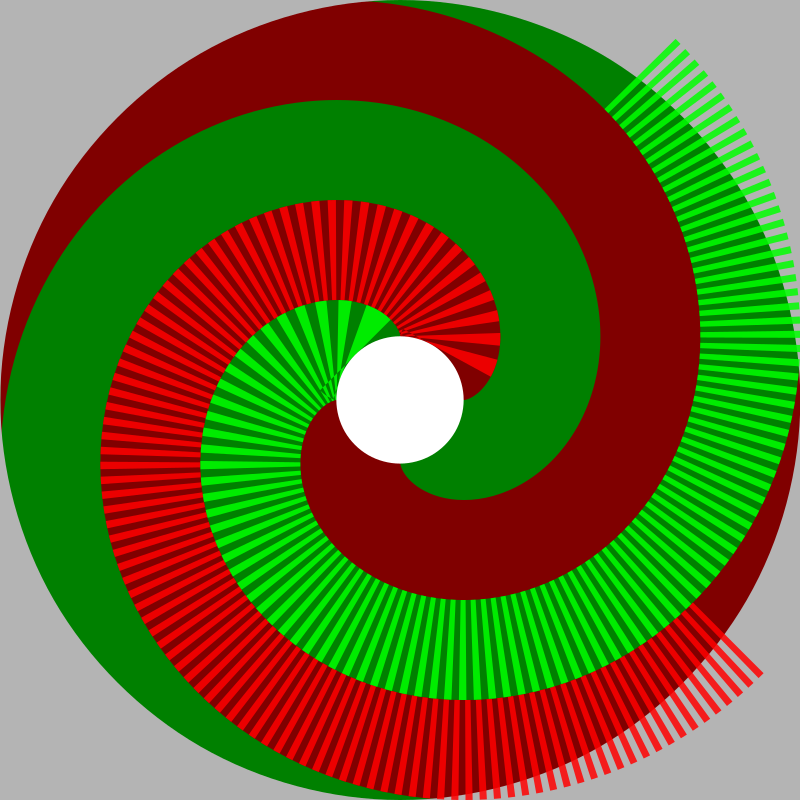## involute of a circle

by Lazur - uploaded on October 11, 2014, 8:51 pm

Probably the right curve was a circle's involute.

"...Its successive turns are parallel curves with constant separation distance..."

Not sure how this can be proven, but this seems to fit well with the concept of stroked path -as parallel curves at it's edges-.

Mathematical functions for the parametric curve is

x=a*(cos(t)+t*sin(t))

y=a*(sin(t)-t*cos(t))